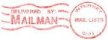McStas: Molecule_2state

# The `Molecule_2state` Component

Disordered optical-excitable molecule sample.

## Identification

• Site:
• Author: Erik B Knudsen
• Origin: DTU Physics
• Date: October 2012

## Description

```A sample model for pump probe experiments which models disordered molecules in a volume (rectangular,
cylindrical, or spherical). Molecules can be in one of two states (0 and 1).
Scattering is either specified through F vs. q scattering curves or as a set of atom positions from which
F vs. q is computed.
At t=-delta_t, a fraction of the molecules are put in state 1, from which they decay exponentially,
with time constant t_relax, into state 0. For t<-delta_t
all of the molecules are in the state specified by initial_state.
To improve statistics, scattering may be limited to a "forward" cone with opening angle in [psimin, psimax].
Furthermore, scattering may be restricted to the azimuthal segment between [etamin,etamax].
```

## Input parameters

Parameters in boldface are required; the others are optional.
 Name Unit Description Default delta_t (s) Delay between the exciting event t=0. delay is negative, i.e. delta_t>0 means the exciting event happens before t=0. 100e-9 excitation_yield ( ) Mean fraction of molecules that get excited. 0.2 t_relax (s) Mean relaxation time (into state 0) of excited molecules. 100e-9 initial_state ( ) Which state is Molecule_2state in for t 0 psimin (rad) Minimum scattering angle off the optical axis. 0 psimax (rad) Maximum scattering angle off the optical axis. M_PI_2 etamin (rad) Minimum scattering angle around the optical axis. -M_PI etamax (rad) Maximum scattering angle around the optical axis. M_PI radius (m) Radius of cylindrical of spherical sample. 0 yheight (m) Height of rectangular or cylindrical sample. 0 xwidth (m) Width of rectangular sample. 0 zdepth (m) Depth (thickness) of rectangular sample. 0 concentration (m) Concentration or packing factor of sample. 1 p_transmit (m) Fraction of statistics devoted to sample direct (unscattered) beam. 0.1 form_factors ( ) File from which to read atomic form factors. Defualt amounts to use the one shipped with McXtrace. "FormFactors.txt" state_0_file ( ) Isotropic scattering factors (parameterized by q), or atom positions are specified for state 0. NULL state_1_file ( ) Isotropic scattering factors (parameterized by q), or atom positions are specified for state 1. NULL nq ( ) Number of q-bins if F is to be computed from atom positions (Debye formalism). 512 material_datafile ( ) Where to read f1 and f2 factors from in order to handle absorption. "Be.txt" q_parametric ( ) 0: Assume that datafiles contains atom positions. 1: datafiles contains F vs. q data. 0 Emax ( ) Maximal energy for which scattering factors are computed. Must be larger than the maximal impinging energy. 80

## Output parameters

Parameters in boldface are required; the others are optional.
 Name Unit Description Default